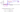## Statistical Classification

The classification problem is just like the regression problem, except that the values we now want to predict take on only a small number of discrete values.

#### Binary classification problem

The value we want to predict $(y)$ can take on only two values, 0 and 1. (Most of what we say here will also generalize to the multiple-class case.) For instance, if we are trying to build a spam classifier for email, then $x^{(i)}$ may be some features of a piece of email, and $y$ may be 1 if it is a piece of spam mail, and 0 otherwise.

Hence, $y \in \{0,1\}$. 0 is also called the negative class, and 1 the positive class, and they are sometimes also denoted by the symbols “-” and “+”.

Given $x^{(i)}$, the corresponding $y^{(i)}$ is also called the label for the training example.

To attempt classification, one method is to use linear regression and map all predictions greater than 0.5 as a 1 and all less than 0.5 as a 0. However, this method doesn't work well because classification is not actually a linear function.If linear regression doesn't work on a classification task as in the above example, applying feature scaling will not make it work.